GATE  >  Electronics And Communication Engineering - (EC) 2018 GATE Paper (Practice Test)

# Electronics And Communication Engineering - (EC) 2018 GATE Paper (Practice Test)

Test Description

## 65 Questions MCQ Test GATE Past Year Papers for Practice (All Branches) | Electronics And Communication Engineering - (EC) 2018 GATE Paper (Practice Test)

Electronics And Communication Engineering - (EC) 2018 GATE Paper (Practice Test) for GATE 2023 is part of GATE Past Year Papers for Practice (All Branches) preparation. The Electronics And Communication Engineering - (EC) 2018 GATE Paper (Practice Test) questions and answers have been prepared according to the GATE exam syllabus.The Electronics And Communication Engineering - (EC) 2018 GATE Paper (Practice Test) MCQs are made for GATE 2023 Exam. Find important definitions, questions, notes, meanings, examples, exercises, MCQs and online tests for Electronics And Communication Engineering - (EC) 2018 GATE Paper (Practice Test) below.
Solutions of Electronics And Communication Engineering - (EC) 2018 GATE Paper (Practice Test) questions in English are available as part of our GATE Past Year Papers for Practice (All Branches) for GATE & Electronics And Communication Engineering - (EC) 2018 GATE Paper (Practice Test) solutions in Hindi for GATE Past Year Papers for Practice (All Branches) course. Download more important topics, notes, lectures and mock test series for GATE Exam by signing up for free. Attempt Electronics And Communication Engineering - (EC) 2018 GATE Paper (Practice Test) | 65 questions in 180 minutes | Mock test for GATE preparation | Free important questions MCQ to study GATE Past Year Papers for Practice (All Branches) for GATE Exam | Download free PDF with solutions
 1 Crore+ students have signed up on EduRev. Have you?
Electronics And Communication Engineering - (EC) 2018 GATE Paper (Practice Test) - Question 1

### “By giving him the last ______ of the cake, you will ensure lasting _____ in our house today.” The words that best fill the blanks in the above sentence are

Electronics And Communication Engineering - (EC) 2018 GATE Paper (Practice Test) - Question 2

### “Even though there is a vast scope for its ________, tourism has remained a/an _________ area.” The words that best fill the blanks in the above sentence are

Electronics And Communication Engineering - (EC) 2018 GATE Paper (Practice Test) - Question 3

### If the number 715∎423 is divisible by 3 (∎ denotes the missing digit in the thousandths place), then the smallest whole number in the place of ∎ is _________.

Electronics And Communication Engineering - (EC) 2018 GATE Paper (Practice Test) - Question 4

What is the value of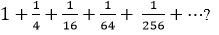Electronics And Communication Engineering - (EC) 2018 GATE Paper (Practice Test) - Question 5

A 1.5 m tall person is standing at a distance of 3 m from a lamp post. The light from the lamp at the top of the post casts her shadow. The length of the shadow is twice her height. What is the height of the lamp post in meters?

Electronics And Communication Engineering - (EC) 2018 GATE Paper (Practice Test) - Question 6

Leila aspires to buy a car worth Rs. 10,00,000 after 5 years. What is the minimum amount in Rupees that she should deposit now in a bank which offers 10% annual rate of interest, if the interest was compounded annually?

Electronics And Communication Engineering - (EC) 2018 GATE Paper (Practice Test) - Question 7

Two alloys A and B contain gold and copper in the ratios of 2:3 and 3:7 by mass, respectively. Equal masses of alloys A and B are melted to make an alloy C. The ratio of gold to copper in alloy C is ______.

Electronics And Communication Engineering - (EC) 2018 GATE Paper (Practice Test) - Question 8

The Cricket Board has long recognized John’s potential as a leader of the team. However, his on-field temper has always been a matter of concern for them since his junior days. While this aggression has filled stadia with die-hard fans, it has taken a toll on his own batting. Until recently, it appeared that he found it difficult to convert his aggression into big scores. Over the past three seasons though, that picture of John has been replaced by a cerebral, calculative and successful batsman-captain. After many years, it appears that the team has finally found a complete captain.

Which of the following statements can be logically inferred from the above paragraph?
(i) Even as a junior cricketer, John was considered a good captain.
(ii) Finding a complete captain is a challenge.
(iii) Fans and the Cricket Board have differing views on what they want in a captain.
(iv) Over the past three seasons John has accumulated big scores.

Electronics And Communication Engineering - (EC) 2018 GATE Paper (Practice Test) - Question 9

A cab was involved in a hit and run accident at night. You are given the following data about the cabs in the city and the accident. (i) 85% of cabs in the city are green and the remaining cabs are blue.
(ii) A witness identified the cab involved in the accident as blue.
(iii) It is known that a witness can correctly identify the cab colour only 80% of the time.
Which of the following options is closest to the probability that the accident was caused by a blue cab?

Electronics And Communication Engineering - (EC) 2018 GATE Paper (Practice Test) - Question 10

A coastal region with unparalleled beauty is home to many species of animals. It is dotted with coral reefs and unspoilt white sandy beaches. It has remained inaccessible to tourists due to poor connectivity and lack of accommodation. A company has spotted the opportunity and is planning to develop a luxury resort with helicopter service to the nearest major city airport. Environmentalists are upset that this would lead to the region becoming crowded and polluted like any other major beach resorts.

Which one of the following statements can be logically inferred from the information given in the above paragraph?

Electronics And Communication Engineering - (EC) 2018 GATE Paper (Practice Test) - Question 11

Two identical nMOS transistors  M1 and M2 are connected as shown below. The circuit is used as an amplifier with the input connected between G and S terminals and the output taken between D and S terminals. Vbias and VD are so adjusted that both transistors are in saturation. The transconductance of this combination is defined as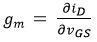while the output
resistance is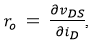where ��D is the current flowing into the drain of M2 . Let gm1 , gm2 be the transconductances and ro1 , ro2 be the output resistances of transistors M1 and M2, respectively.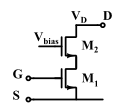Which of the following statements about estimates for gm and ro is correct?

Electronics And Communication Engineering - (EC) 2018 GATE Paper (Practice Test) - Question 12

In the circuit shown below, the op-amp is ideal and Zener voltage of the diode is 2.5 volts. At the input, unit step voltage is applied, i.e. vIN (t) = u(t) volts. Also, at t = 0, the voltage across each of the capacitors is zero.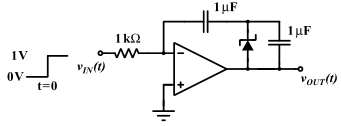The time t, in milliseconds, at which the output voltage vout crosses −10 V is

Electronics And Communication Engineering - (EC) 2018 GATE Paper (Practice Test) - Question 13

A good transimpedance amplifier has

Electronics And Communication Engineering - (EC) 2018 GATE Paper (Practice Test) - Question 14

Let the input be u and the output be y of a system, and the other parameters are real constants. Identify which among the following systems is not a linear system:

Electronics And Communication Engineering - (EC) 2018 GATE Paper (Practice Test) - Question 15

The Nyquist stability criterion and the Routh criterion both are powerful analysis tools for determining the stability of feedback controllers. Identify which of the following statements is FALSE:

Electronics And Communication Engineering - (EC) 2018 GATE Paper (Practice Test) - Question 16

Consider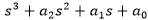with all real coefficients. It is known that its derivative ��′(s) has no real roots. The number of real roots of ��(s) is

Electronics And Communication Engineering - (EC) 2018 GATE Paper (Practice Test) - Question 17

In a p-n junction diode at equilibrium, which one of the following statements is NOT TRUE?

Electronics And Communication Engineering - (EC) 2018 GATE Paper (Practice Test) - Question 18

The logic function f ( X , Y ) realized by the given circuit is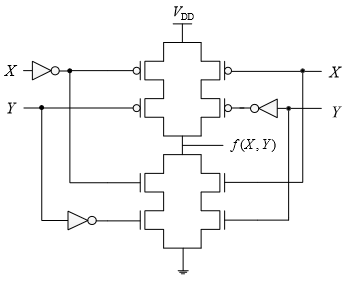Electronics And Communication Engineering - (EC) 2018 GATE Paper (Practice Test) - Question 19

A function F(A, B, C ) defined by three Boolean variables A, B and C when expressed as sum of products is given by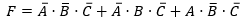where,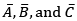are the complements of the respective variables. The product of sums (POS) form of the function F is

Electronics And Communication Engineering - (EC) 2018 GATE Paper (Practice Test) - Question 20

The points P, Q, and R shown on the Smith chart (normalized impedance chart) in the following figure represent: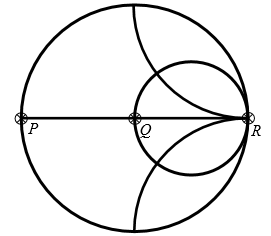Electronics And Communication Engineering - (EC) 2018 GATE Paper (Practice Test) - Question 21

Let M be a real 4x4 matrix. Consider the following statements:
S1:  M has 4 linearly independent eigenvectors.
S2:  M has 4 distinct eigenvalues.
S3:  M is non-singular (invertible).
Which one among the following is TRUE?

Electronics And Communication Engineering - (EC) 2018 GATE Paper (Practice Test) - Question 22

Let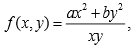where a and b are constants. If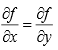at x = 1 and y= 2 , then the relation between a and b is

Electronics And Communication Engineering - (EC) 2018 GATE Paper (Practice Test) - Question 23

A discrete-time all-pass system has two of its poles at 0.25∠0 and 2∠30 . Which one of the following statements about the system is TRUE?

Electronics And Communication Engineering - (EC) 2018 GATE Paper (Practice Test) - Question 24

Let x(t) be a periodic function with period T = 10. The Fourier series coefficients for this series are denoted by ak, that is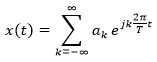The same function  x(t) can also be considered as a periodic function with period T ′ = 40. Let bk be the Fourier series coefficients when period is taken as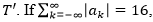then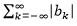is equal to

Electronics And Communication Engineering - (EC) 2018 GATE Paper (Practice Test) - Question 25

Consider the following amplitude modulated signal: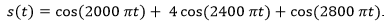The ratio (accurate to three decimal places) of the power of the message signal to the power of the carrier signal is __________.

Electronics And Communication Engineering - (EC) 2018 GATE Paper (Practice Test) - Question 26

Consider a binary channel code in which each codeword has a fixed length of 5 bits. The Hamming distance between any pair of distinct codewords in this code is at least 2. The maximum number of codewords such a code can contain is _________.

Electronics And Communication Engineering - (EC) 2018 GATE Paper (Practice Test) - Question 27

A binary source generates symbols x ∈ {−1,1} which are transmitted over a noisy channel. The probability of transmitting x = 1 is 0.5. Input to the threshold detector is R = X + ��.
The probability density function fN (n) of the noise �� is shown below.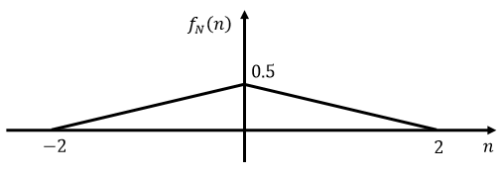If the detection threshold is zero, then the probability of error (correct to two decimal places) is __________.

Electronics And Communication Engineering - (EC) 2018 GATE Paper (Practice Test) - Question 28

A p-n step junction diode with a contact potential of 0.65 V has a depletion width of 1 μm at equilibrium. The forward voltage (in volts, correct to two decimal places) at which this width reduces to 0.6 μm is   _______.

Electronics And Communication Engineering - (EC) 2018 GATE Paper (Practice Test) - Question 29

A traffic signal cycles from GREEN to YELLOW, YELLOW to RED and RED to GREEN. In each cycle, GREEN is turned on for 70 seconds, YELLOW is turned on for 5 seconds and the RED is turned on for 75 seconds. This traffic light has to be implemented using a finite state machine (FSM). The only input to this FSM is a clock of 5 second period. The minimum number of flip-flops required to implement this FSM is   _______.

Electronics And Communication Engineering - (EC) 2018 GATE Paper (Practice Test) - Question 30

There are two photolithography systems: one with light source of wavelength λ1 = 156 nm (System 1) and another with light source of wavelength λ2 = 325 nm (System 2).  Both photolithography systems are otherwise identical.  If the minimum feature sizes that can be realized using System1 and System2 are Lmin1 and Lmin2 respectively, the ratio Lmin1/Lmin2 (correct to two decimal places) is__________.

Electronics And Communication Engineering - (EC) 2018 GATE Paper (Practice Test) - Question 31

A lossy transmission line has resistance per unit length R = 0.05Ω / m . The line is distortionless and has characteristic impedance of 50Ω . The attenuation constant (in Np/m, correct to three decimal places) of the line is _______.

Electronics And Communication Engineering - (EC) 2018 GATE Paper (Practice Test) - Question 32

Consider matrix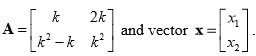. The number of distinct real values
of k for which the equation Ax = 0 has infinitely many solutions is _______.

Electronics And Communication Engineering - (EC) 2018 GATE Paper (Practice Test) - Question 33

Let X 1 , X 2 , X 3 and X 4 be independent normal random variables with zero mean and unit variance. The probability that X 4 is the smallest among the four is _______.

Electronics And Communication Engineering - (EC) 2018 GATE Paper (Practice Test) - Question 34

Taylor series expansion of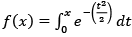around x = 0 has the form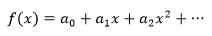The coefficient a2 (correct to two decimal places) is equal to _______.

Electronics And Communication Engineering - (EC) 2018 GATE Paper (Practice Test) - Question 35

The ABCD matrix for a two-port network is defined by: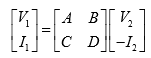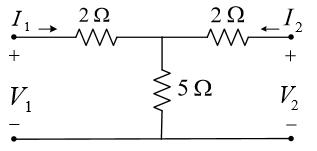The parameter B for the given two-port network (in ohms, correct to two decimal places) is _______.

Electronics And Communication Engineering - (EC) 2018 GATE Paper (Practice Test) - Question 36

The circuit shown in the figure is used to provide regulated voltage (5 V) across the 1kΩ resistor. Assume that the Zener diode has a constant reverse breakdown voltage for a current range, starting from a minimum required Zener current, I Z min = 2 mA to its maximum allowable current. The input voltage VI may vary by 5% from its nominal value of 6 V. The resistance of the diode in the breakdown region is negligibl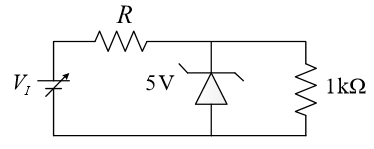Electronics And Communication Engineering - (EC) 2018 GATE Paper (Practice Test) - Question 37

Let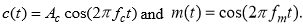. It is given that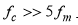The signal c(t ) + m(t ) is applied to the input of a non-linear device, whose output vo (t) is related to the input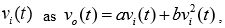where a and b are positive constants. The output of the non-linear device is passed through an ideal band-pass filter with center frequency f c and bandwidth 3 f m , to produce an amplitude modulated (AM) wave. If it is desired to have the sideband power of the AM wave to be half of the carrier power, then ab is

Electronics And Communication Engineering - (EC) 2018 GATE Paper (Practice Test) - Question 38

Consider a white Gaussian noise process ��(t) with two-sided power spectral density SN(f) = 0.5 W/Hz as input to a filter with impulse response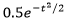(where t is in seconds) resulting in output y(t). The power in y(t) in watts is

Electronics And Communication Engineering - (EC) 2018 GATE Paper (Practice Test) - Question 39

The state equation and the output equation of a control system are given below: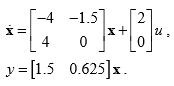The transfer function representation of the system is

Electronics And Communication Engineering - (EC) 2018 GATE Paper (Practice Test) - Question 40

Red (R), Green (G) and Blue (B) Light Emitting Diodes (LEDs) were fabricated using p-n junctions of three different inorganic semiconductors having different band-gaps. The builtin voltages of red, green and blue diodes are VR, VG and VB, respectively.  Assume donor and acceptor doping to be the same (NA and ND, respectively) in the p and n sides of all the three diodes. Which one of the following relationships about the built-in voltages is TRUE?

Electronics And Communication Engineering - (EC) 2018 GATE Paper (Practice Test) - Question 41

A four-variable Boolean function is realized using 4x1 multiplexers as shown in the figure.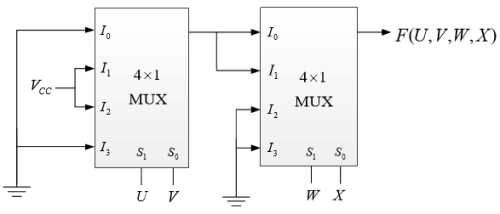The minimized expression for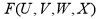is

Electronics And Communication Engineering - (EC) 2018 GATE Paper (Practice Test) - Question 42

A 2 x 2 ROM array is built with the help of diodes as shown in the circuit below.  Here W0 and W1 are signals that select the word lines and B0 and B1 are signals that are output of the sense amps based on the stored data corresponding to the bit lines during the read operation.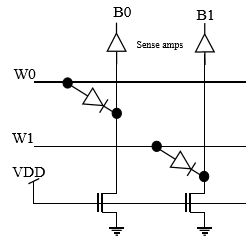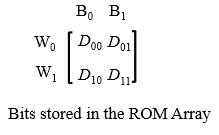During the read operation, the selected word line goes high and the other word line is in a high impedance state.  As per the implementation shown in the circuit diagram above, what are the bits corresponding to Dij (where i = 0 or 1 and j = 0 or 1) stored in the ROM?

Electronics And Communication Engineering - (EC) 2018 GATE Paper (Practice Test) - Question 43

The distance (in meters) a wave has to propagate in a medium having a skin depth of 0.1 m so that the amplitude of the wave attenuates by 20 dB, is

Electronics And Communication Engineering - (EC) 2018 GATE Paper (Practice Test) - Question 44

A curve passes through the point ( x = 1,y= 0 ) and satisfies the differential equation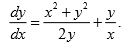The equation that describes the curve is

Electronics And Communication Engineering - (EC) 2018 GATE Paper (Practice Test) - Question 45

For the circuit given in the figure, the voltage VC (in volts) across the capacitor is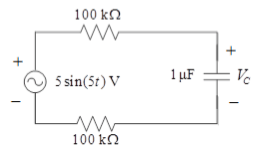Electronics And Communication Engineering - (EC) 2018 GATE Paper (Practice Test) - Question 46

For the circuit given in the figure, the magnitude of the loop current (in amperes, correct to three decimal places) 0.5 second after closing the switch is _______.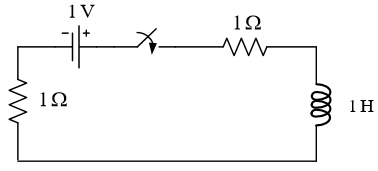Electronics And Communication Engineering - (EC) 2018 GATE Paper (Practice Test) - Question 47

A dc current of 26 μA flows through the circuit shown. The diode in the circuit is forward biased and it has an ideality factor of one. At the quiescent point, the diode has a junction capacitance of 0.5 nF . Its neutral region resistances can be neglected. Assume that the room temperature thermal equivalent voltage is 26 mV.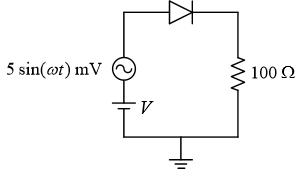For w = 2x106 rad/s , the amplitude of the small-signal component of diode current (in μA, correct to one decimal place) is _______.

Electronics And Communication Engineering - (EC) 2018 GATE Paper (Practice Test) - Question 48

An op-amp based circuit is implemented as shown below.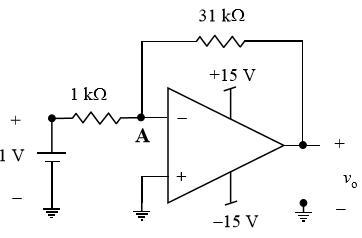In the above circuit, assume the op-amp to be ideal. The voltage (in volts, correct to one decimal place) at node A, connected to the negative input of the op-amp as indicated in the figure is _________.

Electronics And Communication Engineering - (EC) 2018 GATE Paper (Practice Test) - Question 49

The input 4sinc(2t) is fed to a Hilbert transformer to obtain y(t), as shown in the figure below: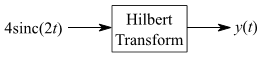Here sinc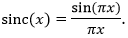The value (accurate to two decimal places) of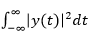is   _______.

Electronics And Communication Engineering - (EC) 2018 GATE Paper (Practice Test) - Question 50

A random variable X takes values −0.5 and 0.5 with probabilities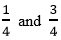, respectively. The noisy observation of X is Y = X + Z, where Z has uniform probability density over the interval (−1, 1). X and Z are independent. If the MAP rule based detector outputs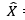as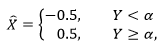then the value of �� (accurate to two decimal places) is _______.

Electronics And Communication Engineering - (EC) 2018 GATE Paper (Practice Test) - Question 51

For a unity feedback control system with the forward path transfer function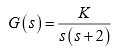The peak resonant magnitude M r of the closed-loop frequency response is 2. The corresponding value of the gain K (correct to two decimal places) is _________.

Electronics And Communication Engineering - (EC) 2018 GATE Paper (Practice Test) - Question 52

The figure below shows the Bode magnitude and phase plots of a stable transfer function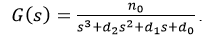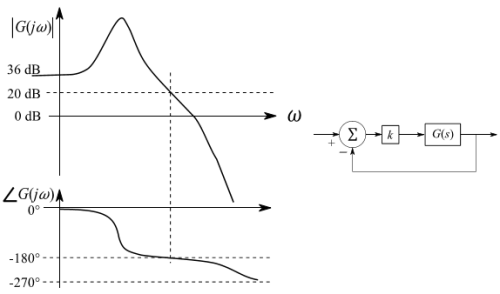Consider the negative unity feedback configuration with gain k in the feedforward path.
The closed loop is stable for k < k0 . The maximum value of k0 is ______.

Electronics And Communication Engineering - (EC) 2018 GATE Paper (Practice Test) - Question 53

In the circuit shown below, the (W/L) value for M2 is twice that for M1. The two nMOS transistors are otherwise identical. The threshold voltage VT for both transistors is 1.0V. Note that VGS for M2 must be > 1.0 V.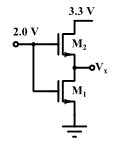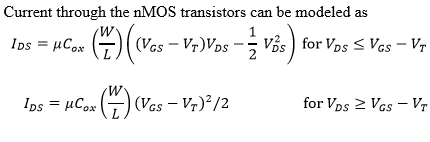The voltage (in volts, accurate to two decimal places) at Vx is  _______.

Electronics And Communication Engineering - (EC) 2018 GATE Paper (Practice Test) - Question 54

A solar cell of area 1.0 cm 2 , operating at 1.0 sun intensity, has a short circuit current of 20 mA, and an open circuit voltage of 0.65 V. Assuming room temperature operation and thermal equivalent voltage of 26 mV, the open circuit voltage (in volts, correct to two decimal places) at 0.2 sun intensity is _______.

Electronics And Communication Engineering - (EC) 2018 GATE Paper (Practice Test) - Question 55

A junction is made between p- Si with doping density NA1 = 1015 cm-3 and p Si with doping density NA2 = 1017 cm-3
Given: Boltzmann constant k = 1.38 x 10-23 J×K-1, electronic charge q = 1.6 x 10-19 C. Assume 100% acceptor ionization.
At room temperature (T = 300K), the magnitude of the built-in potential (in volts, correct to two decimal places) across this junction will be _________________.

Electronics And Communication Engineering - (EC) 2018 GATE Paper (Practice Test) - Question 56

In the circuit shown below, a positive edge-triggered D Flip-Flop is used for sampling input data Din using clock CK. The XOR gate outputs 3.3 volts for logic HIGH and 0 volts for logic LOW levels. The data bit and clock periods are equal and the value of  ΔT/TCK = 0.15, where the parameters ΔT and TCK are shown in the figure. Assume that the Flip-Flop and the XOR gate are ideal.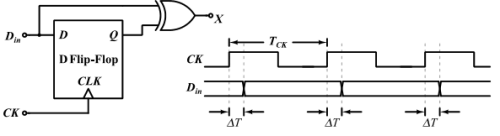If the probability of input data bit (Din) transition in each clock period is 0.3, the average value (in volts, accurate to two decimal places) of the voltage at node X, is   _______.

Electronics And Communication Engineering - (EC) 2018 GATE Paper (Practice Test) - Question 57

The logic gates shown in the digital circuit below use strong pull-down nMOS transistors for LOW logic level at the outputs. When the pull-downs are off, high-value resistors set the output logic levels to HIGH (i.e. the pull-ups are weak). Note that some nodes are intentionally shorted to implement “wired logic”. Such shorted nodes will be HIGH only if the outputs of all the gates whose outputs are shorted are HIGH.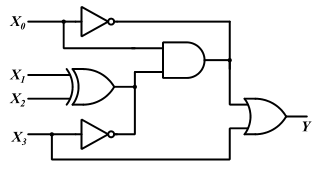The number of distinct values of X3X2X1X0 (out of the 16 possible values) that give X = 1 is   _______.

Electronics And Communication Engineering - (EC) 2018 GATE Paper (Practice Test) - Question 58

The cutoff frequency of TE01 mode of an air filled rectangular waveguide having inner dimensions a cm x b cm ( a >b ) is twice that of the dominant TE10 mode. When the waveguide is operated at a frequency which is 25% higher than the cutoff frequency of the dominant mode, the guide wavelength is found to be 4 cm. The value of b (in cm, correct to two decimal places)  is _______.

Electronics And Communication Engineering - (EC) 2018 GATE Paper (Practice Test) - Question 59

A uniform plane wave traveling in free space and having the electric field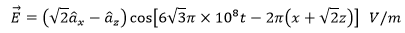is incident on a dielectric medium (relative permittivity > 1, relative permeability = 1) as shown in the figure and there is no reflected wave.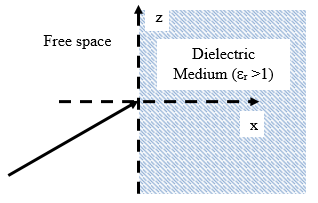The relative permittivity (correct to two decimal places) of the dielectric medium is

Electronics And Communication Engineering - (EC) 2018 GATE Paper (Practice Test) - Question 60

The position of a particle y(t) is described by the differential equation: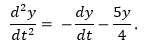The initial conditions are  The position (accurate to two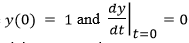decimal places) of the particle at t = �� is   _______.

Electronics And Communication Engineering - (EC) 2018 GATE Paper (Practice Test) - Question 61

The contour C given below is on the complex plane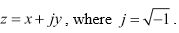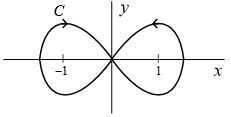The value of the integral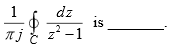Electronics And Communication Engineering - (EC) 2018 GATE Paper (Practice Test) - Question 62

Let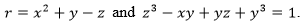. Assume that x and y are independent variables. At (x, y, z) = (2, −1,1), the value (correct to two decimal places) of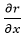is
_________ .

Electronics And Communication Engineering - (EC) 2018 GATE Paper (Practice Test) - Question 63

Consider the network shown below with R1 = 1Ω, R2 = 2Ω and R3 = 3Ω. The network is connected to a constant voltage source of 11V.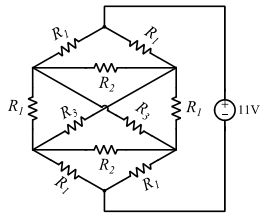The magnitude of the current (in amperes, accurate to two decimal places) through the source is   _______

Electronics And Communication Engineering - (EC) 2018 GATE Paper (Practice Test) - Question 64

A band limited low-pass signal x(t) of bandwidth 5 kHz is sampled at a sampling rate fs . The signal x(t) is reconstructed using the reconstruction filter H(f) whose magnitude response is shown below: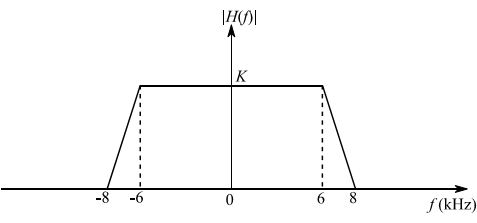The minimum sampling rate fs (in kHz) for perfect reconstruction of x(t) is _______.

Electronics And Communication Engineering - (EC) 2018 GATE Paper (Practice Test) - Question 65

Let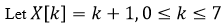be 8-point DFT of a sequence x [n],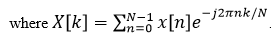The value (correct to two decimal places) of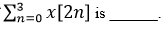## GATE Past Year Papers for Practice (All Branches)

407 docs|127 tests
 Use Code STAYHOME200 and get INR 200 additional OFF Use Coupon Code
Information about Electronics And Communication Engineering - (EC) 2018 GATE Paper (Practice Test) Page
In this test you can find the Exam questions for Electronics And Communication Engineering - (EC) 2018 GATE Paper (Practice Test) solved & explained in the simplest way possible. Besides giving Questions and answers for Electronics And Communication Engineering - (EC) 2018 GATE Paper (Practice Test), EduRev gives you an ample number of Online tests for practice

## GATE Past Year Papers for Practice (All Branches)

407 docs|127 tests